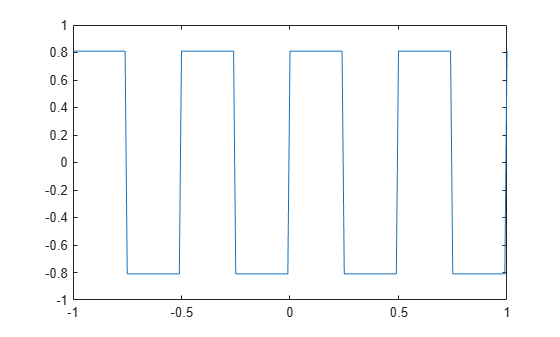# Impulse, Step, and Ramp Functions

Since MATLAB® is a programming language, an endless variety of different signals is possible. Here are some statements that generate a unit impulse, a unit step, a unit ramp, and a unit parabola.

```t = (-1:0.01:1)'; impulse = t==0; unitstep = t>=0; ramp = t.*unitstep; quad = t.^2.*unitstep;```

All of these sequences are column vectors that inherit their shapes from `t`. Plot the sequences.

`plot(t,[impulse unitstep ramp quad])`Generate and plot a square wave with period 0.5 and amplitude 0.81.

```sqwave = 0.81*square(4*pi*t); plot(t,sqwave)```